The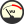SAR Sensor computes the unaveraged specific absorption rate (SAR) that determines the interaction between electromagnetic fields and human tissue. Most wireless communications devices operating between 30 MHz and 6 GHz must not exceed specified exposure limits, typically defined in terms of the peak spatial-average specific absorption rate over a cubical volume of tissue, called voxels.

## Background

SAR has units of watts per kilogram ($W/kg$) and is defined

\begin{equation} SAR = \frac{\sigma |E|^2}{\rho} \end{equation}

Where $\sigma$ is the electrical conductivity of the tissue in siemens per meter ($S/m$), $\rho$ is the mass density of the tissue in kilograms per cubic meter ($kg/m^3$), and $E$ is the peak electric field vector in volts per meter ($V/m$). $\sigma$ and $\rho$ for a part are specified through the part's material definition.

Because the FDTD grid defines the electric fields at the cell edges, the SAR value for a single voxel is formed by summing and averaging the contributions of the 12 electric fields on the associated cell edges. SAR is then referenced to the center of the FDTD cell. SAR is computed only in normal dielectric or debye-drude materials. Other electric material types are not supported for SAR calculations.

The SAR values are saved only in closed FDTD cells, called complete voxels, where the 12 cell edges are lossy dielectric material with a non-zero density. Therefore, SAR values do not exist in all planes. Users can exclude certain materials from a SAR calculation by entering zero as the material density. Saving SAR in a plane of free-space does not produce useful output because all of the values are zero.

Users should note that most specifications that involve SAR limits are defined in terms of constant-mass regions, and therefore require averaged SAR.

## Controls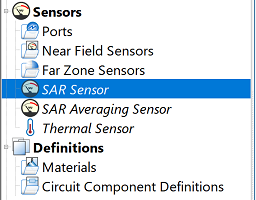Users can enable a SAR sensor by double-clicking on SAR Sensor in the Project Tree to open the editor, and then checking the Enable Collection of Raw SAR Data option. Users should note that the SAR sensor will automatically be enabled and set up by XF when the SAR averaging sensor is used.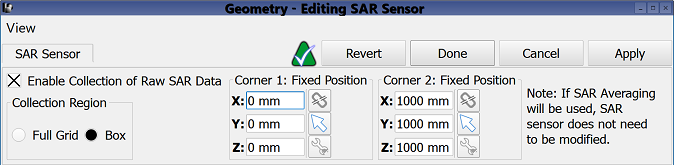Requesting raw SAR data enables the Collection Region section, which defines where SAR will be computed. The Full Grid option collects SAR data within the entire FDTD space. The Box option collects SAR data within a specified bounding box, which is defined using the adjacent Corner 1 and Corner 2 settings.

These options allow users to manually enter coordinates using the X, Y, and Z spaces provided, or use the picker tools (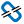,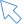,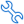) directly to the right of them. From top to bottom, the buttons detach the point from the geometry it is attached to, select a reference point on existing geometry, and access advanced options.